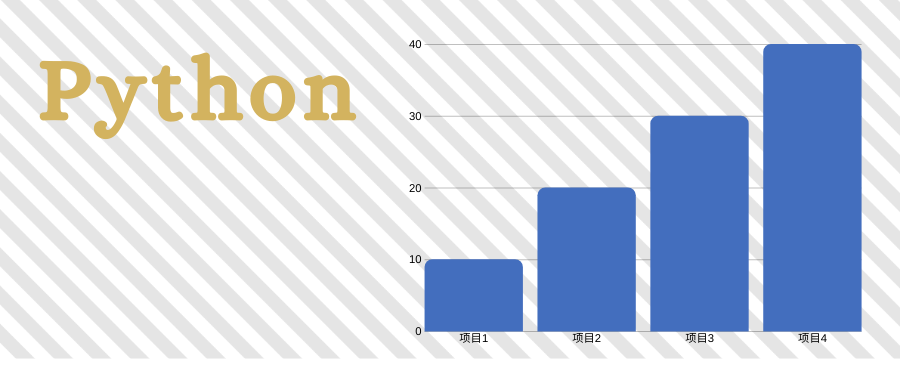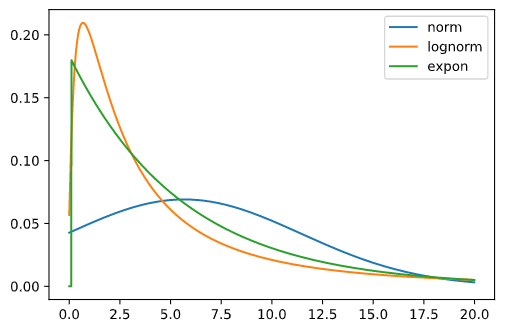# 如何通过样本数据推断其分布# 2. 分析

• Bayesian information criterion

• Kolmogorov–Smirnov test

• Cramér–von Mises criterion

• Anderson–Darling test

• Shapiro–Wilk test

• Chi-squared test

Scipy 的统计模块 stats，可以做 ks 测试。但有个问题是，分布参数需要我们提供。比如指数分布，其概率密度函数import scipy.stats as statsimport numpy as npimport matplotlib.pyplot as pltdata = np.loadtxt('data.txt', delimiter=',', encoding='utf8')dists = {'norm': stats.norm, 'lognorm': stats.lognorm, 'expon': stats.expon}for d in dists:    paras = dists[d].fit(data)    test = stats.kstest(data, dists[d].cdf, paras)    print('{:1}\tpvalue:{:2}'.format(d, test[-1]))

norm	pvalue:0.18025230160179895lognorm	pvalue:0.703843693432498expon	pvalue:0.705389895669437

x = np.arange(0, 20, 0.01)paras = stats.norm.fit(data)ynorm = stats.norm.pdf(x, paras, paras)paras = stats.lognorm.fit(data)ylognorm = stats.lognorm.pdf(x, paras, paras, paras)paras = stats.expon.fit(data)yexpon = stats.expon.pdf(x, paras, paras)fig, ax = plt.subplots()ax.plot(x, ynorm, label='norm')ax.plot(x, ylognorm, label='lognorm')ax.plot(x, yexpon, label='expon')ax.legend()# 4. 交流Lily

# 6. 参考文献

1. W. McKinney, Python for Data Analysis. Beijing: O’Reilly, 2017.

2. J. VanderPlas, Python Data Science Handbook. Beijing: O’Reilly, 2016.Data2Science## 评论